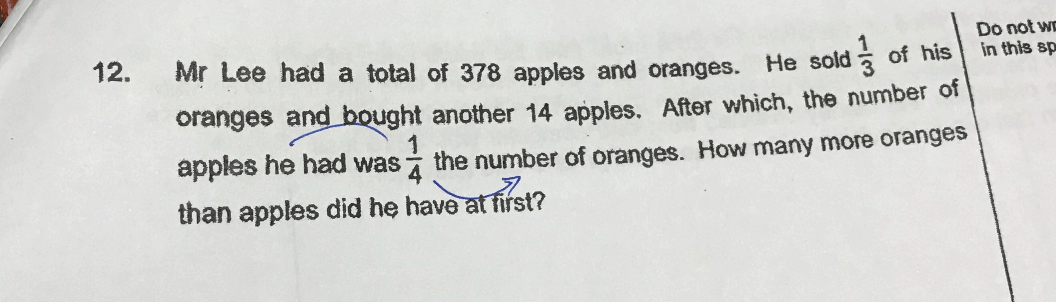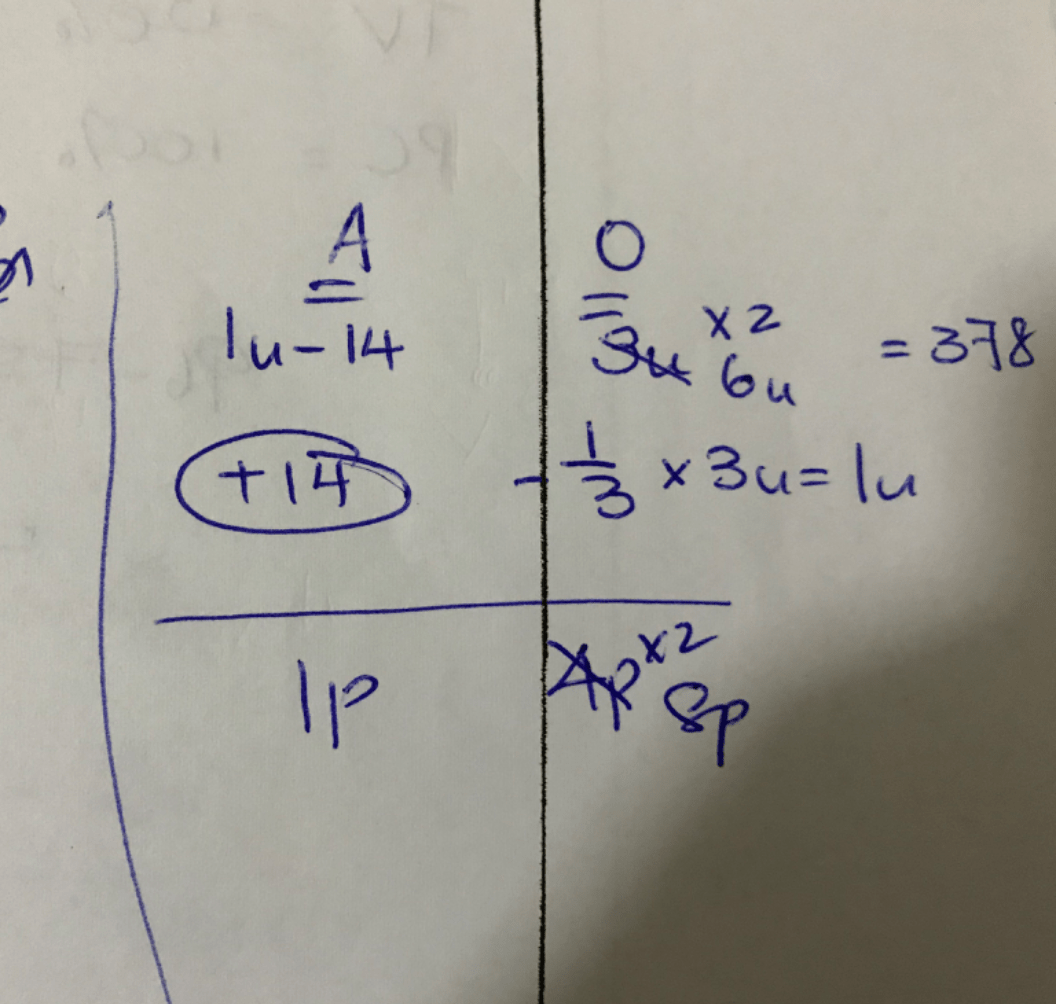# QuestionHi

need some guide to the above question.

the given answer is 294 which I couldn’t get it.

appreciate any guide and help.

Source: Nan Hua Primary

2 Answers

# Answer

Method 1 :
oranges : 3 units – 1 units = 2 units
apples : (378 – 3 units) + 14 = 392 – 3 units

392 – 3 units = 2 units/4
1568 – 12 units = 2 units
1 unit = 1568/(2 + 12) = 112
3 x 112 – (378 – 3 x 112) = 336 – (378 – 336) = 294

Method 2 :
oranges :
3u – 1u = 2u ——-> 4p
1u ——-> 2p

apples :
(378 – 3u) + 14 = 392 – 3u ——-> 1p
784 – 6u ——-> 2p

1u ——-> 784 – 6u
1u ——-> 784/(1 + 6) = 112
3u – (378 – 3u) = 3 x 112 – (378 – 3 x 112) = 294

Ans : 294 more oranges.

1 Reply 0 Likes ✔Accepted AnswerCan I express such way and do I need to change the apples?

1 Reply 0 Likes

You have made a number of mistakes using units and parts. My solution, using units and parts, is as follows :

Method 2 :
oranges :
3u – 1u = 2u ——-> 4p
1u ——-> 2p

apples :
(378 – 3u) + 14 = 392 – 3u ——-> 1p
784 – 6u ——-> 2p

1u ——-> 784 – 6u
1u ——-> 784/(1 + 6) = 112
3u – (378 – 3u) = 3 x 112 – (378 – 3 x 112) = 294

1 Reply 0 Likes

Thanks .

noted my mistakes.

Appreciate that.

1 Reply 0 Likes

You are most welcome.

0 Replies 0 Likes